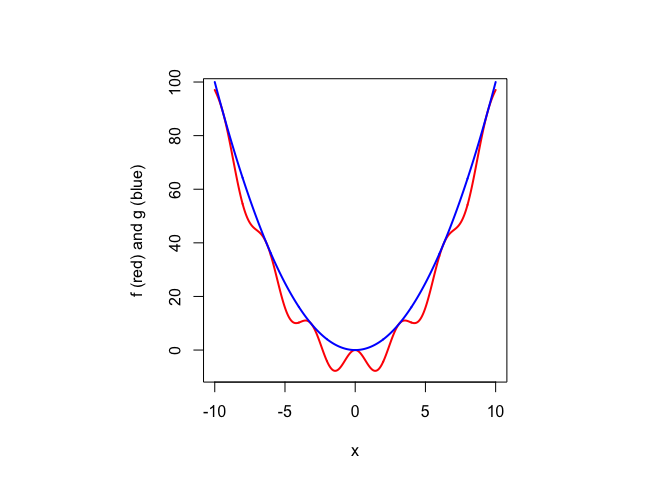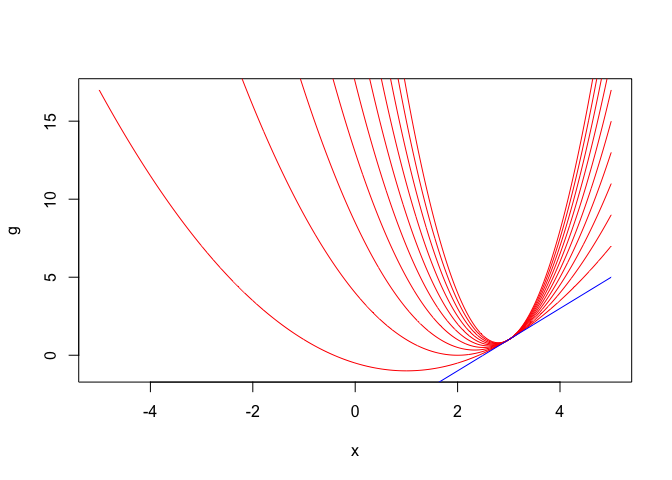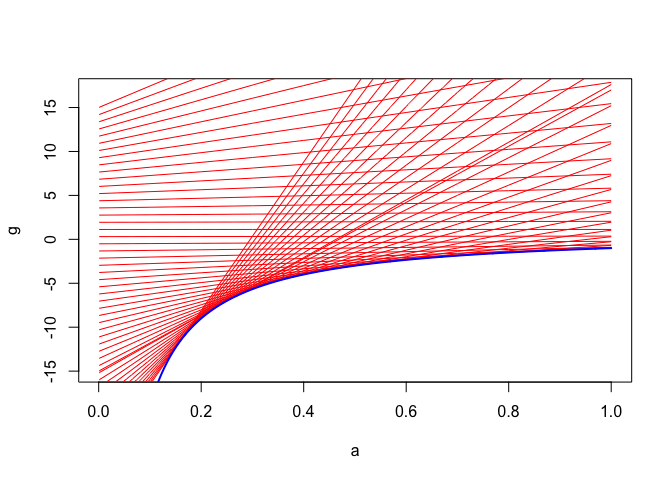Note: This is a working paper which will be expanded/updated frequently. All suggestions for improvement are welcome. The directory deleeuwpdx.net/pubfolders/fans has a pdf version, the bib file, the complete Rmd file, and the R and C code (if applicable).

# 1 Introduction

Majorization algorithms are popular these days. The basic idea is simple. To minimize a real valued target function $$f:\mathcal{S}\Rightarrow\mathbb{R}$$ on a set $$\mathcal{S}\subseteq\mathbb{R}^n$$ we use an iterative algorithm in which iteration $$k+1$$ updates $$x^{(k)}\in\mathcal{S}$$ to $$x^{(k+1)}\in\mathcal{S}$$ in two substeps. In the first substep we find a function $$g$$ that lies above the target function in $$\mathcal{S}$$ and touches it in the current $$x^{(k)}$$. In the second substep we find $$x^{(k+1)}$$ by minimizing the majorizing function $$g$$ over $$\mathcal{S}$$. This produces a strictly decreasing sequence of target function values $$f^{(k)}=f(x^{(k)})$$, which forces convergence under some natural additional conditions (D’Esopo (1959), Zangwill (1969)).

Early majorization algorithms for specific classes of problems were described by Dempster, Laird, and Rubin (1977) and De Leeuw (1977). Both papers suggest that a general class of algorithms lies behind their proposals. As a natural next step, some more general families of majorization methods were discussed in Vosz and Eckhardt (1980) and Böhning and Lindsay (1988). A general theory, inspired by both Dempster, Laird, and Rubin (1977) and De Leeuw (1977), was introduced in De Leeuw (1994) and Heiser (1995), and a much improved and expanded version is now available in book form in Lange (2016) and De Leeuw (2016).

Minimizing $$f$$ is done by constructing majorizations $$g$$. But of course we can also maximize $$f$$ by using minorizers $$g$$. Thus Lange and co-workers (for example Hunter and Lange (2004)) defined the class of MM algorithms, which cleverly covers both majorization-minimization and minorization-maximization. In this paper we only talk about majorization-minimization, because it is trivial to switch from one to the other anyway (by using $$-f$$ and $$-g$$).

Now for notation.

• The real numbers are $$\mathbb{R}$$, the positive reals are $$\mathbb{R}_+$$, and the vector space of $$n$$-tuples of real numbers is $$\mathbb{R}^n$$. The extended reals (with $$\pm\infty)$$ are $$\overline{\mathbb{R}}$$.

• I have already used the notation $$f:\mathcal{X}\Rightarrow\mathcal{Y}$$ for a function from $$\mathcal{X}$$ to $$\mathcal{Y}$$. If $$f:\mathcal{X}\otimes\mathcal{Y}\Rightarrow\mathcal{Z}$$ then $$f(\bullet,y):\mathcal{X}\Rightarrow\mathcal{Z}$$ for each $$y\in\mathcal{Y}$$. Thus $$f(\bullet,y)(x)=f(x,y)$$. If $$f(x)=\|x\|$$, for example, I also use the notation $$\|\bullet\|$$ for the function $$f$$. Throughout I try to distinguish between the function and the values it takes, so I avoid saying “the function f(x)=ax+b”.

• Successive derivatives of $$f:\mathcal{X}\Rightarrow\mathcal{Y}$$ are $$\mathcal{D}f, \mathcal{D}^2f,$$ and so on. If the domain $$\mathcal{X}$$ is a subset of the real line $$\mathbb{R}$$ we also use $$f', f'', f''', f^{iv},$$ and so on. If $$g:\mathcal{X}\otimes\mathcal{Y}\Rightarrow\mathcal{Z}$$ we use $$\mathcal{D}_1g, \mathcal{D}_2g, \mathcal{D}_{11}g=\mathcal{D}_1\mathcal{D}_1g$$ and so on. See Spivak (1965), p. 44-45.

• The symbol $$\mathop{=}^\Delta$$ is used for definitions.

• End of proof is $$\blacksquare$$.

# 2 Majorization Basics

Definition 1: Suppose $$f:\mathcal{S}\Rightarrow\mathbb{R}$$ and $$g:\mathcal{S}\Rightarrow\mathbb{R}$$. Then we say $$g$$ majorizes $$f$$ on $$\mathcal{S}$$ if $$g(x)\geq f(x)$$ for all $$x\in\mathcal{S}$$. If $$\mathcal{S}$$ is all of $$\mathbb{R}^n$$ we usually leave out the “on $$\mathcal{S}$$”.

Example 1: If $$f:\mathbb{R}\Rightarrow\mathbb{R}$$ is a non-trivial cubic and $$g:\mathbb{R}\Rightarrow\mathbb{R}$$ is a quadratic, then $$g$$ does not majorize $$f$$ on $$\mathbb{R}$$ and $$f$$ does not majorize $$g$$ on $$\mathbb{R}$$. Majorization of $$f$$ by $$g$$ would imply $$g(x)-f(x)\geq 0$$ for all $$x\in\mathbb{R}$$, and $$g-f$$ is a non-trivial cubic, which cannot be non-negative on the whole line. Similar for majorization of $$g$$ by $$f$$.

Definition 2: If $$g$$ majorizes $$f$$ on $$\mathcal{S}$$ then the support set of the majorization is the set of all $$x\in\mathcal{S}$$ with $$g(x)=f(x)$$. Thus for $$x\in\mathcal{S}$$ not in the support set we have $$g(x)>f(x)$$. Elements of the support set are support points.

Note 1: We usually abbreviate “$$g$$ majorizes $$f$$ on $$\mathcal{S}$$ with $$y\in\mathcal{S}$$ a support point of the majorization” to “$$g$$ majorizes $$f$$ on $$\mathcal{S}$$ at $$y$$”.

Note 2: The set $$\mathcal{G}$$ of all functions majorizing $$f$$ on $$\mathcal{S}$$ is convex. If we order $$\mathcal{G}$$ using majorization and define functions $$g\wedge h$$ and $$g\vee h$$ by $$(g\wedge h)(x)=\min(g(x),h(x))$$ and $$(g\vee h)(x)=\max(g(x),h(x))$$ then $$\mathcal{G}$$ becomes an inf-complete lattice. Both the convexity and the lattice property remain true for the set $$\mathcal{G}_Y$$ of all functions majorizing $$f$$ on $$\mathcal{S}$$ with a given support set $$Y$$. Both $$G$$ and $$G_Y$$ have as their unique minimum element the function $$f$$.

Example 2: There can be zero, one, a finite number, or an infinite number of support points of a majorization. The example in figure 1, from De Leeuw and Lange (2009), has $$g(x)=x^2$$ and $$f(x)=x^2-10\sin^2(x)$$. The support set are all integer multiples of $$\pi$$.Figure 1: Support Set

Definition 3: A strict majorization on $$\mathcal{S}$$ at $$y$$ is a majorization with a unique support point.

Note 3: If $$f$$ is convex, then $$g$$ with $$g(x)=f(y)+z'(x-y)$$ majorizes $$f$$ at $$y$$, for any $$z$$ in the subgradient $$\partial f(y)$$. If $$f$$ is strictly convex the majorization is strict.

Note 4: If $$f$$ is two times differentiable, and there is a $$B$$ such that $$B-\mathcal{D}^2f(x)$$ is positive semi-definite for all $$x$$ then $$g$$ with $$g(x)=f(y)+(x-y)'\mathcal{D}f(y)+\frac12(x-y)'B(x-y)$$ majorizes $$f$$ at $$y$$.

Note 5: If a differentiable $$g$$ majorizes a differentiable $$f$$ on $$\mathbb{R}$$ then $$\mathcal{D}f(y)=\mathcal{D}g(y)$$ at any support point. If a twice differentiable $$g$$ majorizes a twice differentiable $$f$$ on $$\mathbb{R}$$ then $$\mathcal{D}^2g(y)\succeq\mathcal{D}^2f(y)$$ at any support point, i.e. $$\mathcal{D}^2g(y)-\mathcal{D}^2f(y)$$ is positive semi-definite. This is because the differentiable function $$h=g-f$$ has a minimum, equal to zero, at any support point. If majorization is strict we have $$\mathcal{D}^2g(y)\succ\mathcal{D}^2f(y)$$.

# 3 Majorization Algorithm

Definition 4: A majorization algorithm is an iterative algorithm intended to minimize $$f$$ over $$x\in\mathcal{S}$$. Iteration $$k$$ starts with a current $$x^{(k)}\in\mathcal{S}$$. Select a $$g$$ that majorizes $$f$$ on $$\mathcal{S}$$ at $$x^{(k)}$$ and find $$x^{(k+1)}\in\mathcal{S}$$ such that $$g(x^{(k+1)})<g(x^{(k)})$$. If there is no $$x\in\mathcal{S}$$ with $$g(x)<g(x^{(k)})$$ the algorithm stops.

The key to why majorization algorithms work (i.e. converge) is the following result.

Theorem 1: Suppose $$g$$ majorizes $$f$$ on $$\mathcal{S}$$ at $$y$$. Then, for all $$x\in\mathcal{S}$$, $$g(x)<g(y)$$ implies $$f(x)<f(y)$$.

Proof: $$f(x)\leq g(x)$$ by majorization, $$g(x)<g(y)$$ by assumption, and $$g(y)=f(y)$$ by support. Thus we have the sandwich inequality $$f(x)\leq g(x)<g(y)=f(y)$$. If majorization is strict this becomes $$f(x)< g(x)<g(y)=f(y)$$. But even if $$x$$ is a second support point of the majorization we still have $$f(x)=g(x)<g(y)=f(y)$$. $$\blacksquare$$

Note 6: Definition 4 does not tell us how to select majorizations. In that sense it is an incomplete definition, which makes it impossible to study the properties of the algorithm. To actually get an implementation going, we need a more complete definition.

Definition 5: A majorization scheme for $$f:\mathcal{S}\Rightarrow\mathbb{R}$$ on $$\mathcal{S}$$ is a function $$g:\mathcal{S}\times\mathcal{S}\Rightarrow\mathbb{R}$$ such that

• $$g(x,y)\geq f(x)$$ for all $$x,y\in\mathcal{S}$$,
• $$g(x,x) = f(x)$$ for all $$x\in\mathcal{S}$$.

In other words, for each $$y\in\mathcal{S}$$ the function $$g(\bullet,y)$$ majorizes $$f$$ on $$\mathcal{S}$$ at $$y$$.

Definition 6: [Redone] Suppose $$g$$ is a majorization scheme for $$f$$ on $$\mathcal{S}$$. In a majorization algorithm iteration $$k$$ starts with a current $$x^{(k)}\in\mathcal{S}$$. We then choose $$x^{(k+1)}\in\mathcal{S}$$ such that $$g(x^{(k+1)},x^{(k)})<g(x^{(k)},x^{(k)})$$. The sandwich inequality becomes $f^{(k+1)}\leq g(x^{(k+1)},x^{(k)})<g(x^{(k)},x^{(k)})<f(x^{(k)}).$ If there is no $$x\in\mathcal{S}$$ with $$g(x,x^{(k)})<g(x^{(k)},x^{(k)})$$ the algorithm stops.

Note 8: Not every majorization scheme leads to a useful majorization algorithm. The function $$g$$ with $$g(x,y)=f(y)+\alpha|x-y|$$ is a majorization scheme for $$f$$ for any $$\alpha>0$$. But it is impossible to choose $$x^{(k+1)}$$ such that $$g(x^{(k+1)},x^{(k)})<g(x^{(k)},x^{(k)})$$, so the algorithm stops immediately at any initial solution $$x^{(0)}$$.

# 4 Fans of Functions

Definition 7: A fan on $$\mathcal{S}$$ at $$y\in\mathcal{S}$$ is a function $$g:\mathcal{S}\otimes\mathcal{A}\Rightarrow\mathbb{R}$$, with $$\mathcal{A}$$ a real interval, such that

• $$g(y,\bullet)$$ is a constant function,
• If $$\alpha<\beta$$ then $$g(x,\alpha)<g(x,\beta)$$ for all $$x\not= y$$.

Thus for $$\alpha<\beta$$ we have $$g(\bullet,\beta)$$ strictly majorizing $$g(\bullet,\alpha)$$ at $$y$$.

Note 7: Suppose $g(x,0)\mathop{=}^\Delta\inf_{\alpha\in\mathcal{A}} g(x,\alpha)>-\infty.$ Then $$g(\bullet,\alpha)$$ strictly majorizes $$g(\bullet,0)$$ for every $$\alpha\in\mathcal{A}$$.

Note 9: Suppose $$g(\bullet,\alpha)$$ is differentiable at $$y$$ for all $$\alpha$$. Then $$g(\bullet,\beta)-g(\bullet,\alpha)$$ has either a minimum or a maximum at $$y$$, and thus $$\mathcal{D}_1g(y,\alpha)=\mathcal{D}_1g(y,\beta)$$. Thus all $$g(\bullet,\alpha)$$ have the same tangent at $$y$$. If the $$g(\bullet,\alpha)$$ are twice differentiable and $$\alpha<\beta$$ then $$\mathcal{D}_{11}g(y,\alpha)\preceq\mathcal{D}_{11}g(y,\beta)$$ in the Loewner order.

Example 4: Figure 2 is an example of a fan that is quadratic in $$x$$ at $$y=3$$ and linear in $$\alpha\in\mathcal{A}$$, with $g(x,\alpha)=1+2(x-3)+\frac12\alpha(x-3)^2.$ Figure 2 plots the quadratic functions $$g(\bullet,\alpha)$$ for $$\alpha=1,\cdots,10$$. They have their minimum at $$3-2/\alpha$$, with minimum value $$1-2/\alpha$$. The common tangent is the blue line $$g(\bullet,0)=1+2(x-3)$$.Figure 2: Fan, Quadratic in x

The linear functions $$g(x,\bullet)$$ are plotted in figure 3, for $$x$$ taking 50 values between $$-10$$ and $$+10$$. The blue line is the function $$\min_x g(x,\alpha)=1-2/\alpha$$.Figure 3: Fan, Linear in alpha

Example 5: This is a minor modification of example 4. We use $g(x,\alpha)=1+2(x-3)+\frac12\log(\alpha)(x-3)^2.$ This fan is still quadratic in $$x$$, but for $$0<\alpha<1$$ the quadratics are concave. Also for this example there is no $$g(x,0)$$, because $$\inf_{\alpha>0} g(x,\alpha)=-\infty$$ for all $$x\not= 3$$.